# Newton's Laws of Motion - First Law

## Introduction

Sir Isaac Newton published three laws in the 17th century. In this article, we are going to talk about Newton’s 1st law. This law does an introduction of motion of the object and the force acting on it. In other words, it deals with the motion of an object and its relation to force.

## What is Newton’s First Law of Motion?

Newton’s first law states that

a body remains in the state of rest or uniform motion in a straight line unless and until an external force acts on it.

Putting Newton’s 1st law of motion in simple words, a body will not start moving until and unless an external force acts on it. Once it is set in motion, it will not stop or change its velocity until and unless some force acts upon it once more. The first law of motion is sometimes also known as the law of inertia.

There are two conditions on which the 1st law of motion is dependent:

• Objects at rest: When an object is at rest velocity (v= 0) and acceleration (a = 0) are zero. Therefore, the object continues to be at rest.
• Objects in motion: When an object is in motion, velocity is not equal to zero (v ≠ 0) while acceleration (a = 0) is equal to zero. Therefore, the object will continue to be in motion with constant velocity and in the same direction.

Interested to learn how to derive first law of motion, below is the link:

### What is an External Force?

An external force is defined as the change in the mechanical energy that is either the kinetic energy or the potential energy in an object. These forces are caused by external agents. Examples of external forces are friction, normal force and air resistance.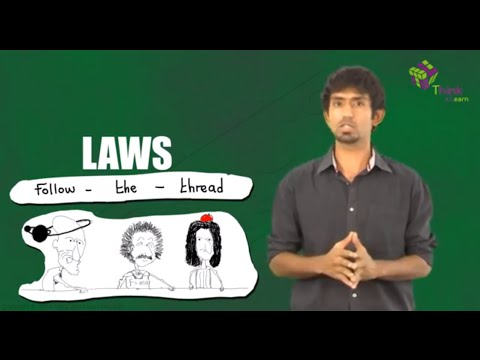### Let us Understand First Law of Motion by an Example

Let us take a block on a smooth surface. By smooth, we mean that there is no friction acting on the surface. The block is at rest, that is, it is not moving.

Now, let ss examine the forces acting on the block. The only forces acting on the block are the force of gravity and the normal reaction by the surface. There is no force acting on it in the horizontal direction. Since the forces in the vertical direction are equal to each other in magnitude, they cancel each other out, and hence there is no external force on the block. Since this block is at rest, we can say that it confirms Newton’s first law of Motion.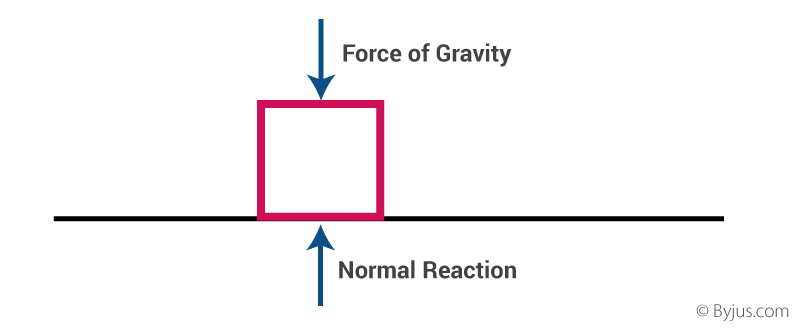Now, if we apply a constant force F on the block in a horizontal direction, it will start moving with some constant acceleration, in the direction of the applied force.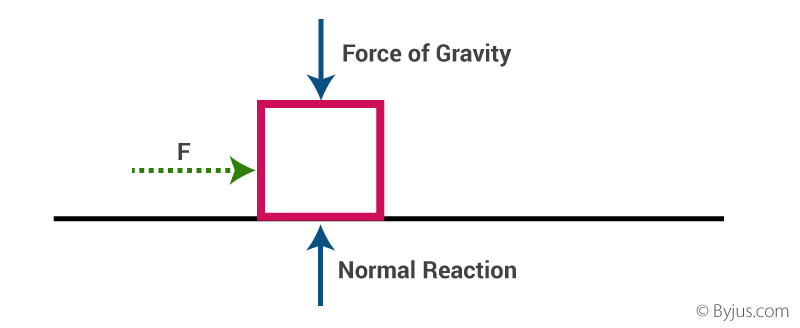Thus, the first law of motion is confirmed again.

Note: Newton’s laws are valid only in inertial frames of reference.

### Newton’s First Law of Motion Examples in Daily Life

Wearing a seat belt in a car while driving is an example of Newton’s 1st law of motion. If an accident occurs, or if brakes are applied to the car suddenly, the body will tend to continue its inertia and move forward, probably proving fatal. To prevent such accidents seat belts are used which stops your body moving forward in inertia avoiding danger.

Below are the links for other laws of motion:

## FAQs on Newton’s First Law of Motion

1. What are the major concepts involved in the topic?

The major concepts involve Frame of reference, Newton’s First law of motion (Law of Inertia), Newton’s Second law of motion, Newton’s Third law of motion (For every action there is an equal and opposite reaction) and constraint equations.

2. What are the characteristics of Normal Force?

The normal force is an electromagnetic force and always acts perpendicular to the surface of contact. For example,

3. What do we understand by Free Body Diagram?

A free body diagram represents all the forces acting on the body. For example,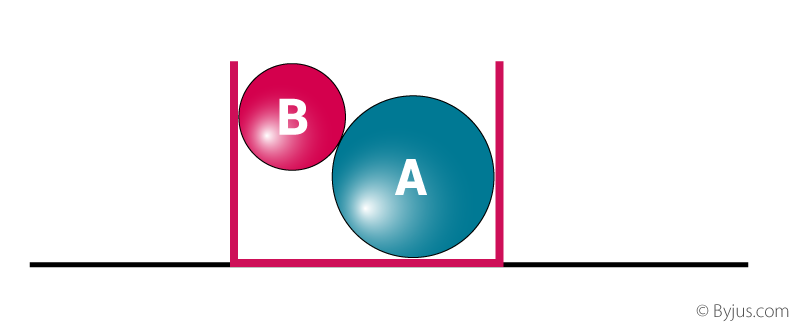So, the FBD for sphere B will be as shown below,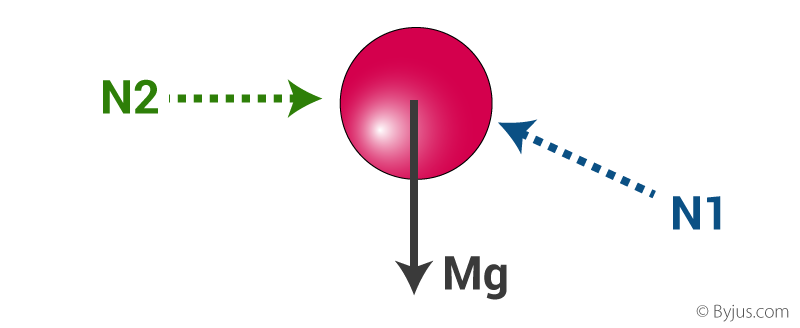4. What are the steps to solve a problem on Newton’s laws of motion?

Let us take the following example,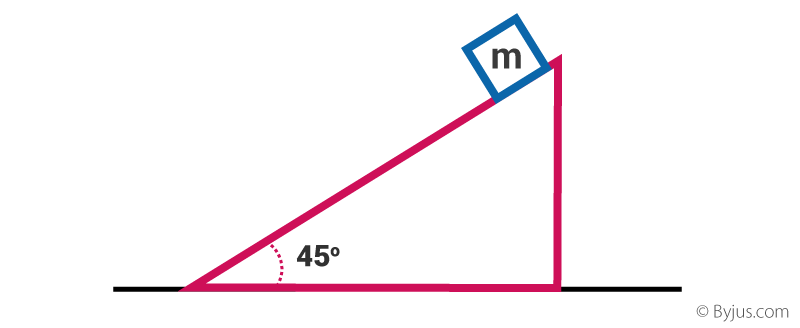The wedge is fixed and we need to find the acceleration of the block of mass m along the incline.

Step 1: Draw the F.B.D of the block,Step 2: Writing the force equation taking our own axis, in this case, we take two axes one along the plane and the other perpendicular to it.

Fincline = $mg~ sin45^{\circ}$

VFNormal = $N ~–~ mg~ cos45^{\circ}$

Step3: Calculating acceleration using second law of motion

$mg ~sin45^{\circ}$  = $ma$

• $a$ = $g~ sin45^{\circ}$

5. What is constraint equation?

When the motion of one body is governed by another or in other words when the motion of two or more bodies is interlinked. The equation governing such motion is known as the constraint equation. For example: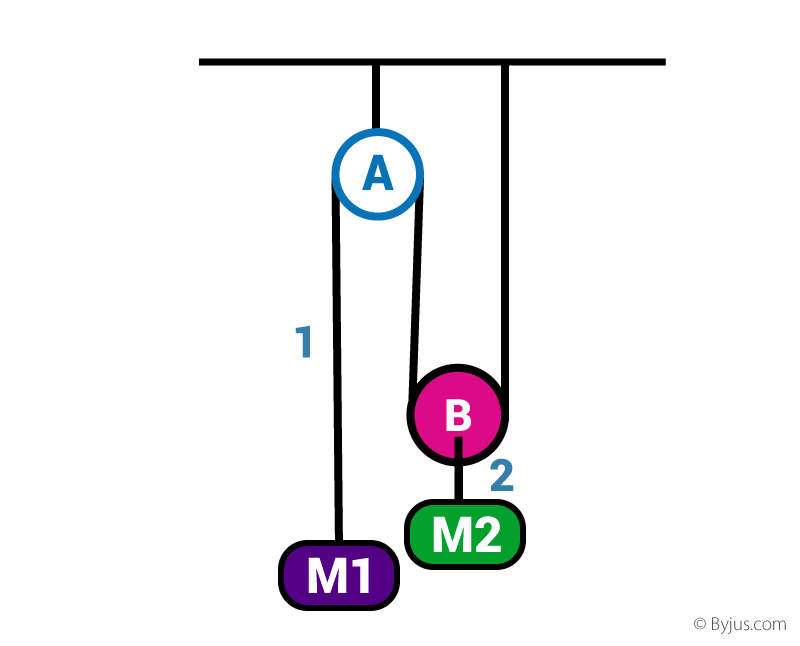If we draw the FBD and write equations we will get,

$M_1.g ~–~ T$ = $M_1.a_1$

$2T – M_2.g$ = $M_2.a_2$

We can see there are two equations and three unknowns: $a_1$, $a_2$ and $T$. So we need one more equation and that will be the constraint equation.

1. vincy

it’s very useful for me

Very nice because this law is very important

3. Bushra

It is nice

4. Mr Why Hurry

It’s very resourceful! Thanks.

5. JR

Car going 60 miles an hour down the road. How fast is the fly flying inside the car and is that also called know?

1. Deepa

Interesting Question! I think the question you mean to ask is whether the fly flies at 60 miles an hour to keep up with the speed of the car?
No, the mosquito doesn’t travel at 60 miles an hour. The fly is inside the car, it is just as much inside the car as you are. Hence, its speed with respect to the car is zero. For an observer standing on a road, the speed of the fly is 60 miles an hour. If the mosquito starts flying forward in the car, it might be doing so with a speed of 1 mph with respect to the car, which is 61 mph with respect to the ground.